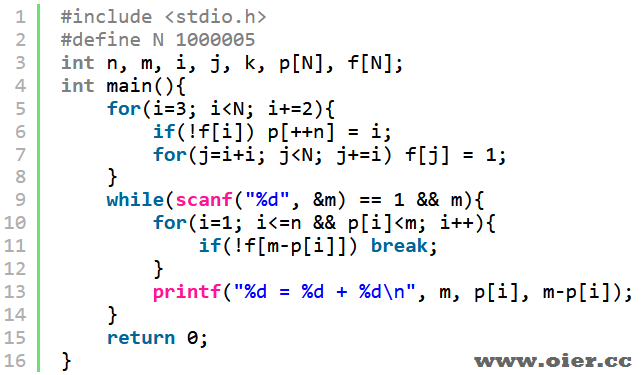SSOJ2902Goldbach
31+

## 题目描述

8=3+520=3+17=7+1342=5+37=11+31=13+29=19+23

## 提示

#### 样例输入

8
20
42
0

#### 样例输出

8 = 3 + 5
20 = 3 + 17
42 = 5 + 37

## 程序实现